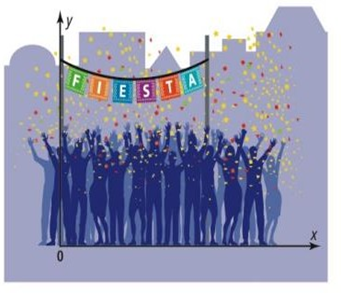Maths-
General
Easy

Question

# A banner is hung for a party . The distance from a point on the bottom edge of the banner to the floor can be determined by using the function f(x)= 0.25x2 -x+9.5, where x is the distance , in feet , of the point from the left end of the banner . How high above the floor is the lowest point on the bottom edge of the banner , Explain.## The correct answer is: 8.5

### Solution:- We have given a function of banner hung.f(x) = 0.25x2 -x+9.5,We have to find the height of lowest point of the bannerThe lowest point will be the vertex of the curve, so the lowest height will be y- coordinate of the vertexOn comparing with the standard form of the function f(x)=ax2+bx+c.In f(x)= 0.25x2 -x+9.5,,  a= 0.25, b= -1, and c=9.5,  So, the equation for the axis of symmetry is given byx = −(-1)/2(0.25)x = 1/0.5x = 2The equation of the axis of symmetry for f(x)= 0.25x2 -x+9.5,  is x = 2.The x coordinate of the vertex is the same:h = 2The y coordinate of the vertex is :k = f(h)k = 0.25h2 -h+9.5,k = 0.25(2)2 -2+9.5,k = 1 - 2 + 9.5k = 8.5Therefore, the vertex is (2 , 8.5)The lowest height will be the y-coordinate of vertex = 8.5#### With Turito Foundation.#### Get an Expert Advice From Turito.